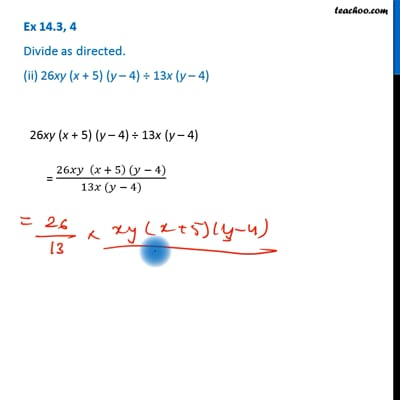Dividing two polynomials

Chapter 12 Class 8 Factorisation
Concept wiseThis video is only available for Teachoo black users

Learn in your speed, with individual attention - Teachoo Maths 1-on-1 Class

### Transcript

Ex 12.3, 4 Divide as directed. (ii) 26xy (x + 5) (y – 4) ÷ 13x (y – 4) 26xy (x + 5) (y – 4) ÷ 13x (y – 4) = (26𝑥𝑦 (𝑥 + 5) (𝑦 − 4))/(13𝑥 (𝑦 − 4)) = (26𝑦 (𝑥 + 5))/13 = 26/13 × y (𝑥 + 5) = 2 × y (𝑥 + 5) = 2y (𝒙 + 𝟓)